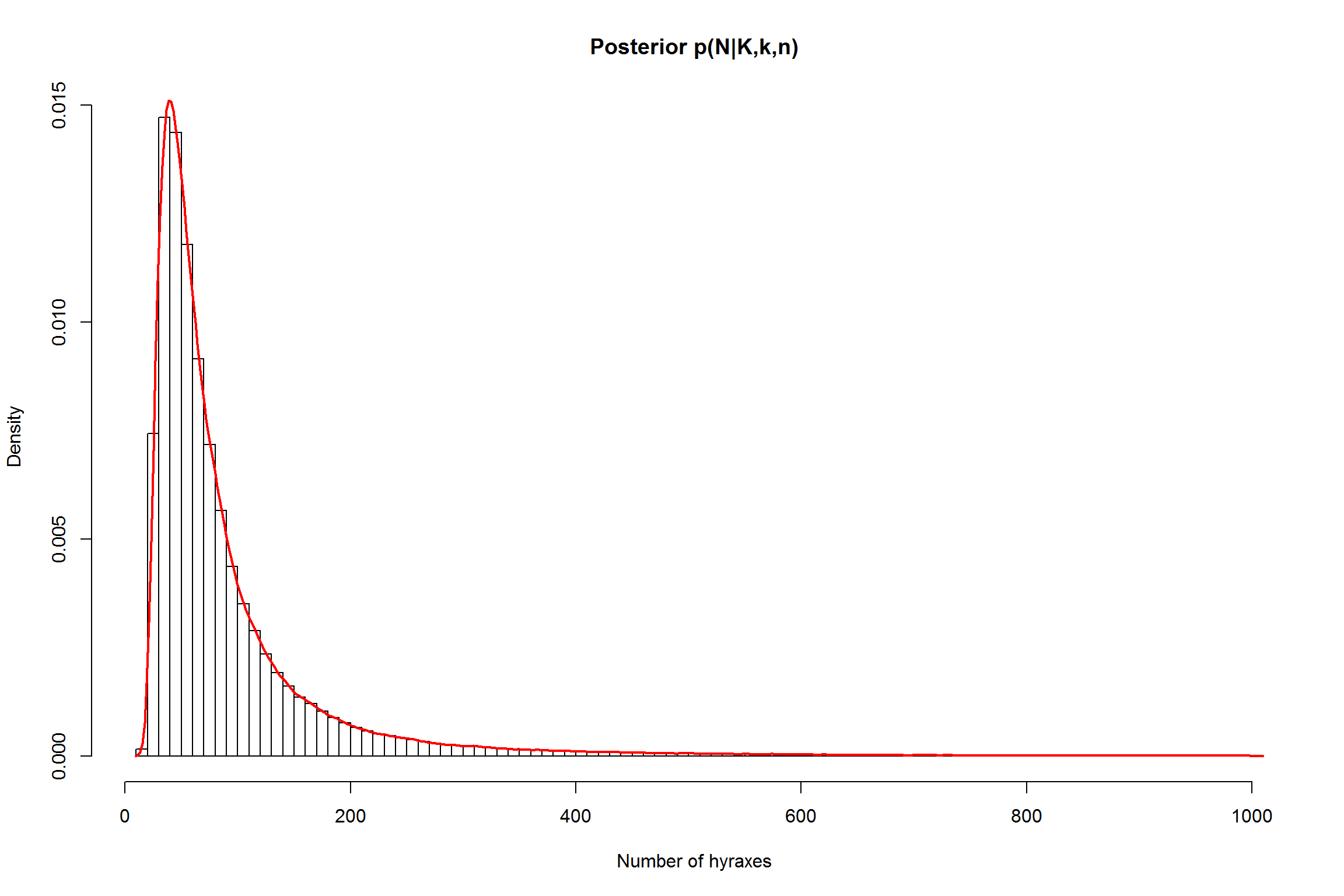Allen Downey proposed the following problem:

Suppose I capture and tag 10 hyraxes. Some time later, I capture another 10 hyraxes and find that two of them are already tagged. How many hyraxes are there in this environment?

We assume the number of hyraxes remain constant, and each hyrax is equally likely to be captured.

Let:

• $$N$$ the total number of hyraxes

• $$K$$ the number of hyraxes tagged in the first capture

• $$n$$ the number of hyraxes captured in the second round

• $$k$$ the number of hyraxes that were tagged in the second round

The likelihood

$p(K,n,k|N) = \frac{{K \choose k}{N-K \choose n-k} }{{N \choose n}}$

check Downey’s solution for details.

Herein I’ll use BUGS to find a solution.

First, my RBugs ‘boilerplate’ code:

library(BRugs)
## Welcome to BRugs connected to OpenBUGS version 3.2.3
run.model <- function(model, samples, data=list(), chainLength=10000, burnin=0.10, init.func, n.chains=1) {

writeLines(model, con="model.txt")  # Write the modelString to a file
modelCheck( "model.txt" )           # Send the model to BUGS, which checks the model syntax
if (length(data)>0)                 # If there's any data available...
modelData(bugsData(data))         # ... BRugs puts it into a file and ships it to BUGS
modelCompile(n.chains)              # BRugs command tells BUGS to compile the model

if (missing(init.func)) {
modelGenInits()                   # BRugs command tells BUGS to randomly initialize a chain
} else {
for (chain in 1:n.chains) {       # otherwise use user's init data
modelInits(bugsInits(init.func))
}
}

modelUpdate(chainLength*burnin)     # Burn-in period to be discarded
samplesSet(samples)                 # BRugs tells BUGS to keep a record of the sampled values
modelUpdate(chainLength)            # BRugs command tells BUGS to randomly initialize a chain
}

So let’s use BUGS to describe this model. I used the ‘Zero’s trick’ since the likelihood function is not standard:

modelString = "
model {

# Likelihood function

phi <- -log(choose1 / choose2) + CZERO
dummy <- 0
dummy ~ dpois( phi )
CZERO <- 10000    # for the zero's trick

# compute binomial coefficients

choose1 <- exp( loggam(N-K+1) - (loggam(n-k+1) + loggam(N-K-(n-k)+1)) ) # choose(N-K,n-k)
choose2 <- exp( loggam(N+1)   - (loggam(n+1)   + loggam(N-n+1)) )       # choose(N,n)

# Priors

N ~ dcat(pN[])  # using jeffrey's prior p(N) propto 1/N

# N ~ dunif(m, M)   # using an uniform prior (too sensitive to M)
}
"

We need to include this data and define some other values for BUGS to run.

To define the prior we need to state a maximum number of hyraxes, $$M$$, which could be properly estimated by the biologists in the field. Placing an uniform prior, however, makes the model too sensitive to $$M$$. In this case, we chose the Jeffrey’s prior

$p(N) \propto \frac{1}{N}$

normalizing it with a given $$M$$:

# data
K <- 10    # the input from the problem
n <- 10
k <- 2
m <- K+n-k # the hyraxes we seen
M <- 1000

p.N <- 1/(1:M)        # making Jeffrey's prior
p.N[1:m] <- 0         # values lower than the hyraxes we already seen are considered impossible
p.N <- p.N / sum(p.N) # normalizing it

# Everything is ready. Run the model!
run.model(modelString, samples=c("N"), data=list(K=K, k=k, n=n, pN=p.N), chainLength=1e5, n.chains=3)
## model is syntactically correct
## data loaded
## model compiled
## initial values generated, model initialized
## 10000 updates took 0 s
## monitor set for variable 'N'
## 100000 updates took 1 s

Let’s vizualize some stats:

# Get stats from the MCMC run
stats <- samplesStats(c("N"))
stats
##   mean    sd MC_error val2.5pc median val97.5pc start sample
## N   91 93.06   0.3508       26     62       353 10001 300000
n.chain <- samplesSample( "N" )    # Extract chain values for number of bugs
dst <- density(n.chain)
map.n.hyraxes <- dst$x[which.max(dst$y)]    # get the MAP from the estimated density
map.n.hyraxes
##  38.81369
# just for vizualization purposes, select the most common values
# n.chain <- n.chain[n.chain<(1.5*stats$val97.5pc)] # Show the posterior distribution for n hist(n.chain, breaks=100, prob=TRUE, xlab="Number of hyraxes", main="Posterior p(N|K,k,n)") lines(dst, col="red", lwd=2)The use of Jeffrey’s prior was to make the model more robust to the upper limit $$M$$. We can test it by running the model with a different value: M <- 500 # instead of 1000 p.N <- 1/(1:M) p.N[1:m] <- 0 p.N <- p.N / sum(p.N) # Everything is ready. Run the model! run.model(modelString, samples=c("N"), data=list(K=K, k=k, n=n, pN=p.N), chainLength=1e5, n.chains=3) ## model is syntactically correct ## data loaded ## model compiled ## initial values generated, model initialized ## 10000 updates took 0 s ## monitor set for variable 'N' ## 100000 updates took 1 s samplesStats(c("N")) ## mean sd MC_error val2.5pc median val97.5pc start sample ## N 84.92 69.54 0.2536 26 62 297 10001 300000 n.chain <- samplesSample( "N" ) # Extract chain values for number of bugs dst <- density(n.chain) map.n.hyraxes <- dst$x[which.max(dst\$y)]    # get the MAP from the estimated density
map.n.hyraxes
##  40.07911

The posterior mean and the map estimate changed but not by that much.